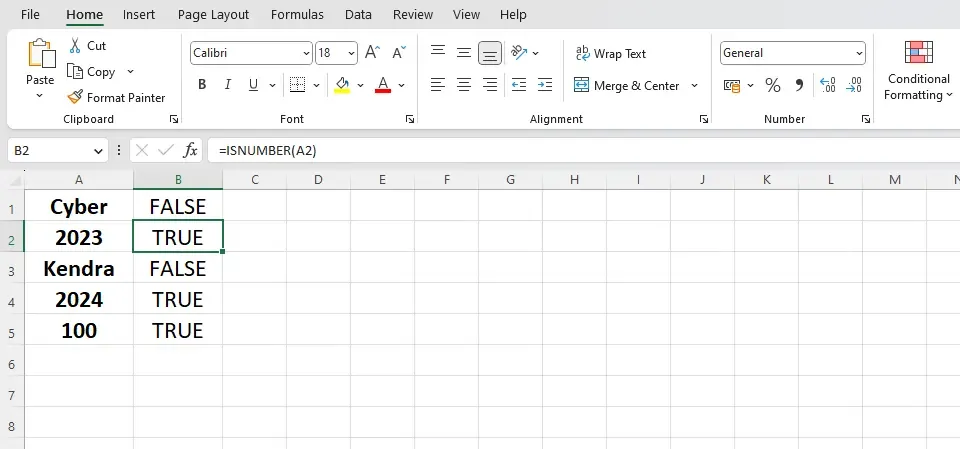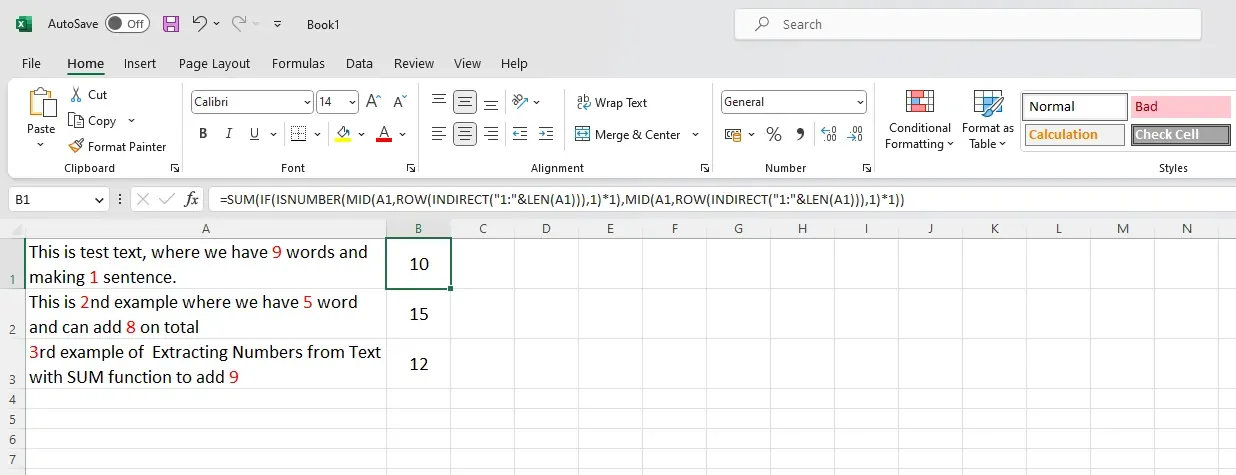# How to Use the ISNUMBER Function in Excel

Master the ISNUMBER function in Excel with our comprehensive guide.Microsoft Excel offers a myriad of powerful functions that aid in analyzing and manipulating data. One such function, the ISNUMBER function, is incredibly useful in identifying whether a cell value is a number or not. In this comprehensive guide, we will explore the ISNUMBER function in-depth, discuss its various applications, and provide practical examples to help you master this essential Excel tool.

The primary function of ISNUMBER is to determine whether a given value is a number or not. This may seem like a simple task, but it is crucial for data validation, error handling, and various analytical tasks. The function returns a Boolean value—TRUE if the input is a number and FALSE if it is not.

## Syntax and Parameters

The ISNUMBER function follows a simple syntax:

`=ISNUMBER(value)`

The parameter 'value' can be a number, a cell reference, a formula, or a text string. The function will analyze the parameter and return the appropriate Boolean value.

### Practical Examples of the ISNUMBER Function

#### A. Validating User Input:

When creating data entry forms, it is important to ensure that users input data in the correct format. The ISNUMBER function can help validate numeric inputs. Consider the following example:
1. In cell A1, type "Enter a number:"
2. In cell B1, create a data validation rule by selecting Data > Data Validation from the Excel menu.
3. In the Data Validation dialog box, select 'Custom' in the 'Allow' dropdown menu.
4. In the 'Formula' field, type "=ISNUMBER(B1)".
5. Click 'OK' to apply the validation rule.If the value in cell A1 is a number, the formula will return TRUE. Otherwise, it will return FALSE.
Now, if a user tries to enter non-numeric data in cell B1, Excel will display an error message and prevent entry.

#### B. Identifying Numeric Cells

The ISNUMBER function can be used to identify cells containing numeric values within a range. This is useful when you need to perform calculations or other operations on numeric data only. For example, consider a range of data in cells A1:A10. To identify which cells contain numbers, you can use the following formula in cell B1 and copy it down through B10:

`=ISNUMBER(A1)`

The resulting TRUE or FALSE values in column B will indicate whether the corresponding cells in column A contain numbers.

## How to Use ISNUMBER with Other Functions

The utilization of the ISNUMBER function in data analysis is a practical and advantageous approach. However, it can be further amplified when utilized in conjunction with other advanced Excel functions. The ISNUMBER function can be combined with other Excel functions to create more complex formulas.

### Examples with Formulas

1. ISNUMBER with SEARCH: The following formula uses ISNUMBER with SEARCH and IF to check if a specific text string is present within a cell and returns the position of the text if found, or "Not Found" if not found:

`=IF(ISNUMBER(SEARCH("text",A1)),SEARCH("text",A1),"Not Found")`

2. ISNUMBER with Conditional Formatting: Conditional formatting can be used to visually highlight numeric values within a range. To apply conditional formatting using ISNUMBER, follow these steps:

1. Select the range of cells you want to apply the formatting.
2. In the Home tab, click 'Conditional Formatting' > 'New Rule'.
3. Choose 'Use a formula to determine which cells to format'.
4. In the 'Format values where this formula is true' field, type "=ISNUMBER(A1)" (replace A1 with the first cell of your selected range).
5. Click 'Format' and choose the desired formatting options.
6. Click 'OK' to apply the conditional formatting.

Now, any numeric values within the selected range will be highlighted with the chosen formatting.

3. Creating Dynamic Charts and Data Validation Lists: ISNUMBER can be used to create dynamic charts or data validation lists that automatically update based on numeric data. For example, consider a list of products with their corresponding prices in cells A1:B10. You can create a dynamic named range that only includes numeric prices using the following steps:

1. Click 'Formulas' > 'Name Manager' > 'New'.
2. In the 'Name' field, type "NumericPrices".
3. In the 'Refers to' field, type "=IF(ISNUMBER(Sheet1!\$B\$1:\$B\$10),Sheet1!\$B\$1:\$B\$10,"")" (replace Sheet1 with the name of your worksheet).
4. Click 'OK' to create the named range.

Now, you can use the named range "NumericPrices" in charts, data validation lists, or other formulas, and it will only include numeric values.

## Tips and Tricks for Using the ISNUMBER Function

a. Checking for Integers or Decimals: To determine if a number is an integer or a decimal, you can combine the ISNUMBER function with the INT function. For example, the following formula will return TRUE if the number in cell A1 is an integer:

`=ISNUMBER(A1) * (A1=INT(A1))`

b. Counting Numeric Cells: If you want to count the number of numeric cells in a range, you can use the ISNUMBER function with the SUMPRODUCT function. For example, to count the number of numeric cells in the range A1:A10, use the following formula:

`=SUMPRODUCT(--ISNUMBER(A1:A10))`

c. Extracting Numbers from Text: You can use the ISNUMBER function with other functions like MID and ROW to extract numbers from a text string. For example, to extract numbers from a text string in cell A1, use the following array formula (press Ctrl + Shift + Enter after typing the formula):

`=SUM(IF(ISNUMBER(MID(A1,ROW(INDIRECT("1:"&LEN(A1))),1)*1),MID(A1,ROW(INDIRECT("1:"&LEN(A1))),1)*1))`

##Frequently Asked Questions

Q: Can I use the ISNUMBER function in Google Sheets?

A: Yes, the ISNUMBER function works similarly in Google Sheets as it does in Excel.

Q: How do I find the last numeric value in a column using ISNUMBER?

A: You can use the following array formula to find the last numeric value in a column (press Ctrl + Shift + Enter after typing the formula):

`=LOOKUP(2,1/(ISNUMBER(A:A)),A:A)`

Replace A:A with the column range you want to search.

## Resources for Further Learning

For more information on the ISNUMBER function and its applications, you can refer to the following resources:

• Microsoft's official documentation on the ISNUMBER function:[LINK]
• ExcelJet's detailed guide on the ISNUMBER function:[LINK]